# Symmetry Class 7 Notes: Chapter 14

According to the CBSE Syllabus 2023-24, this chapter has been renumbered as Chapter 12.

## Basics of Symmetry

• Symmetry is a geometrical concept that is found in most cases including nature.
• Any geometric shape can be said to be symmetric or asymmetric
• A shape is said to be symmetric if there exists an imaginary line passing through that divides the shape into halves and that these halves overlap each other completely.
• In other words, fold the shape about the imaginary line to check if the two halves completely overlap each other or not. If they overlap each other completely the shape is symmetric, if not, then it is asymmetric.
• The imaginary line is called as the line of symmetry.
• The symmetry observed in the above example is called as a line or bilateral symmetry.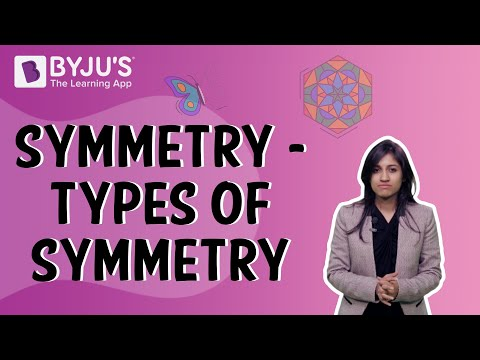To know more about Basics of Symmetry, visit here.

### Reflection Symmetry

• There exists at least one line that divides a figure into two halves such that one-half is the mirror image of the other half.
• Reflection symmetry is a unique case of line symmetry as there exists lateral inversion in the two halves.
• Lateral inversion signifies that left side of one half is the right side of its mirror half.

To know more about Reflection Symmetry, visit here.

### Point Symmetry

• If a shape has point symmetry, then any point on the shape has a matching point which is exactly at the same distance from the point of symmetry but in the opposite direction.

###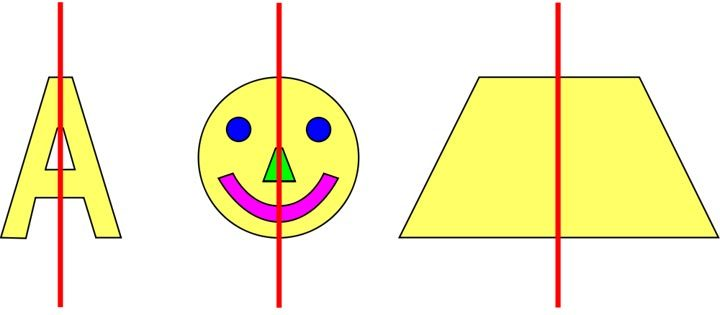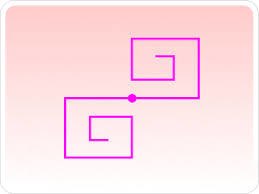## Lines of Symmetry for Regular Polygons

### Lines of symmetry for regular polygons

• Regular polygons are closed shapes that have equal sides and equal angles.
• Such polygons have multiple lines of symmetry.

Some regular polygons with their line of symmetry are shown below: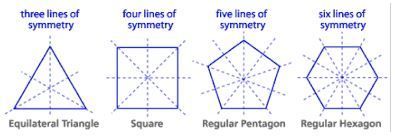To know more about Lines of Symmetry, visit here.

## Rotational Symmetry

• There exists another concept of symmetry that does not involve folding the figure to check if they coincide.
• Rotational symmetry is one where when a figure is rotated about a centre point, the figure looks exactly the same before rotation.
• The particular centre for which rotational symmetry is observed is called the centre of rotation.
• The angle of turning during rotation is called angle of rotation.
• The number of positions in which a figure can be rotated and still appears exactly like it did before the rotation is called order of symmetry.

Rotational symmetry: A figure is rotated around a center point and it still appears exactly like it did before the rotation
Centre of rotation: Fixed point around which the rotation occurs
Angle of rotation: Angle of turning during rotation
Order of Symmetry: Number of positions in which a figure can be rotated and still appears exactly like it did before the rotation
Example: A star can be rotated 5 times along its tip and looks same every time. Hence, its order of symmetry is 5.To know more about Rotational Symmetry, visit here.

### Geometry in rotational symmetry

Rotational symmetry in triangle and square: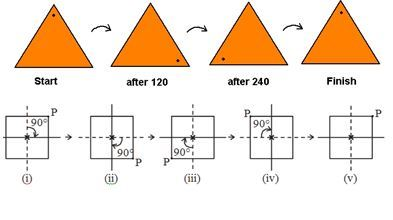## Summary of Symmetry

### Figures with both line symmetry and rotational symmetry

Figures having both line symmetry and rotational symmetry: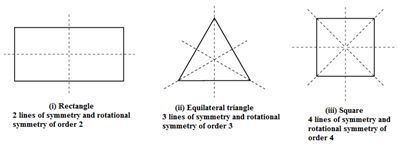Most regular polygons have both line and rotational symmetry.

## Frequently Asked Questions on CBSE Class 7 Maths Notes Chapter 14 Symmetry

Q1

### What is symmetry?

A 2D shape is symmetrical if a line can be drawn through it and either side is a reflection of the other.

Q2

### What are the types of symmetry?

Types of symmetries are rotational symmetry, reflection symmetry, translation symmetry and glide reflection symmetry.

Q3

### What is point symmetry?

Point symmetry occurs when there exists a position or a central point on an object.